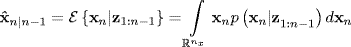# 3.4 Introduction to Recursive Bayesian Estimation of the State Mean and Covariance

Introducing a notation that will be used throughout the remainder of this text, let an estimate of xn conditioned on all observations up to time tp be written aswith

(3.25)Thus, an estimate of xn that uses all observations, including the current one at time tn, is written as

(3.26)while one that predicts xn based on all but the current observation is given by

(3.27)The same can be done for the covariance matrix, writing

(3.28)with prediction form of the covariance given by

(3.29)## 3.4.1 State Vector Prediction

Using the Chapman–Kolmogorov equation (3.24) in (3.27) and (3.29) results in

(3.30)and

From the system dynamic equation (3.17), (3.30) can be rewritten as

In moving from ...

Get Bayesian Estimation and Tracking: A Practical Guide now with the O’Reilly learning platform.

O’Reilly members experience live online training, plus books, videos, and digital content from nearly 200 publishers.